# Test: Synchronous & Asynchronous Circuits

## 10 Questions MCQ Test RRB JE for Computer Science Engineering | Test: Synchronous & Asynchronous Circuits

Description
Attempt Test: Synchronous & Asynchronous Circuits | 10 questions in 30 minutes | Mock test for Computer Science Engineering (CSE) preparation | Free important questions MCQ to study RRB JE for Computer Science Engineering for Computer Science Engineering (CSE) Exam | Download free PDF with solutions
QUESTION: 1

###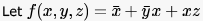be a switching function. Which one of the following is valid?

Solution:

f(x,y,z) = x' + y'x + xz
An implicant of a function is a product term that is included in the function. so x', y'x and xz ,all are implicants of given function.
A prime implicant of a function is an implicant that is not included in any other implicant of the function.
option a)   y'x is not a prime implicant as it is included in xz [ xy'z+ xyz]
option d) y is not a prime implicant as it include in both x' and xz.
a product term in which all the variables appear is called a minterm of the function

QUESTION: 2

### Which are the essential prime implicants of the following Boolean function?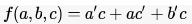Solution:

using K map f = ac' + a'c

QUESTION: 3

### A main memory unit with a capacity of 4 megabytes is built using 1M x 1-bit DRAM chips. Each DRAM chip has 1K rows of cells with 1K cells in each row. The time taken for a single refresh operation is 100 nanoseconds. The time required to perform one refresh operation on all the cells in the memory unit is

Solution:

There are 4*8 = 32 DRAM chips to get 4MB from 1M x 1-bit chips. Now, all chips can be refreshed in parallel so do all cells in a row. So, the total time for refresh will be number of rows times the refresh time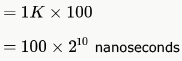*Answer can only contain numeric values
QUESTION: 4

A ROM is used to store the Truth table for a binary multiple unit that will multiply two 4-bit numbers. The size of the ROM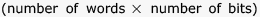that is required to accommodate the Truth table is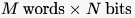. Write the values of M and N.

Solution:

A is 4 bit binary no A4A3A2A1
B is 4 bit binary no B4B3B2B1
M is result of multiplication M8M7M6M5M4M3M2M1     [check biggest no 1111 x 1111 =11100001]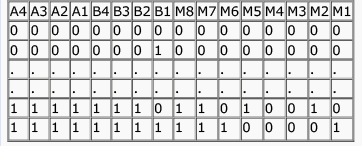So 4 bit of A 4 bit of B
input will consist of 8 bit need address 00000000 to 11111111 = 28 address
output will be of 8 bits
so memory will be of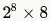M = 256 , N = 8

QUESTION: 5

A ROM is used to store the table for multiplication of two 8-bit unsigned integers. The size of ROM required is

Solution:

When we multiply two 8 bit numbers result can go up to 16 bits. So, we need 16 bits for each of the multiplication result.
Number of results possible = 28 ⨯ 28 = 216 = 64 K as we need to store all possible results of multiplying two 8 bit numbers. So, 64 K ⨯ 16 is the answer.

QUESTION: 6

What is the minimum size of ROM required to store the complete truth table of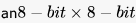multiplier?

Solution:

multiplying 2 8 bit digits will give result in maximum 16 bits
total number of multiplications possible = 28 x 28
hence space required = 64K x 16 bits

QUESTION: 7

The amount of ROM needed to implement a 4 - bit  multiplier is

Solution:

A ROM cannot be written. So, to implement a 4 bit multiplier we must store all the possible combinations of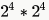input bits and  output bits giving a total of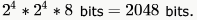. So, (D) is the answer.

QUESTION: 8

Which of the following input sequences for a cross-coupled R-S flip-flop realized with two NAND gates may lead to an oscillation?

Solution:

For R-S flip flop with NAND gates (inputs are active low) 11-no change 00-indeterminate..............so option A may make the system oscillate as "00" is the final input. In option D, after "00" flipflop output may oscillate but after "11", it will be stabilized.

QUESTION: 9

The below figure shows four D-type flip-flops connnected as a shift register using an XOR gate. The initial state and three subsequent states for three clock pulses are also given.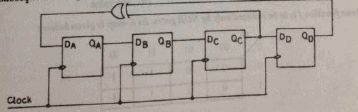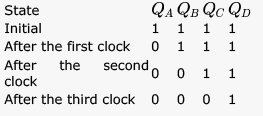The state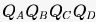after the fourth clock pulse is

Solution: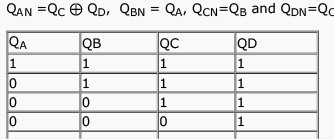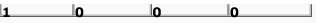QUESTION: 10

Consider the circuit given below with initial state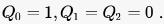. The state of the circuit is given by the value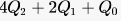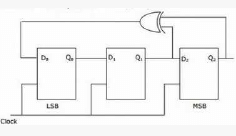Which one of the following is correct state sequence of the circuit?

Solution: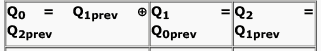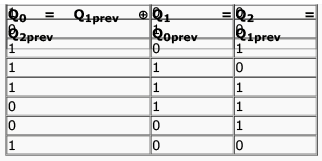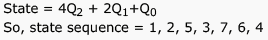Use Code STAYHOME200 and get INR 200 additional OFF Use Coupon Code